# Improving pgRouting Performance

#### The Network

I've loaded up the new Open Roads network dataset from Ordnance Survey into PostGIS and got it working with pgRouting. I can route successfully from A to B across the network. The problem is that it is so slow. There are 3.18 million road links and 2.9 million nodes in the UK network and it takes a long time to solve the problem.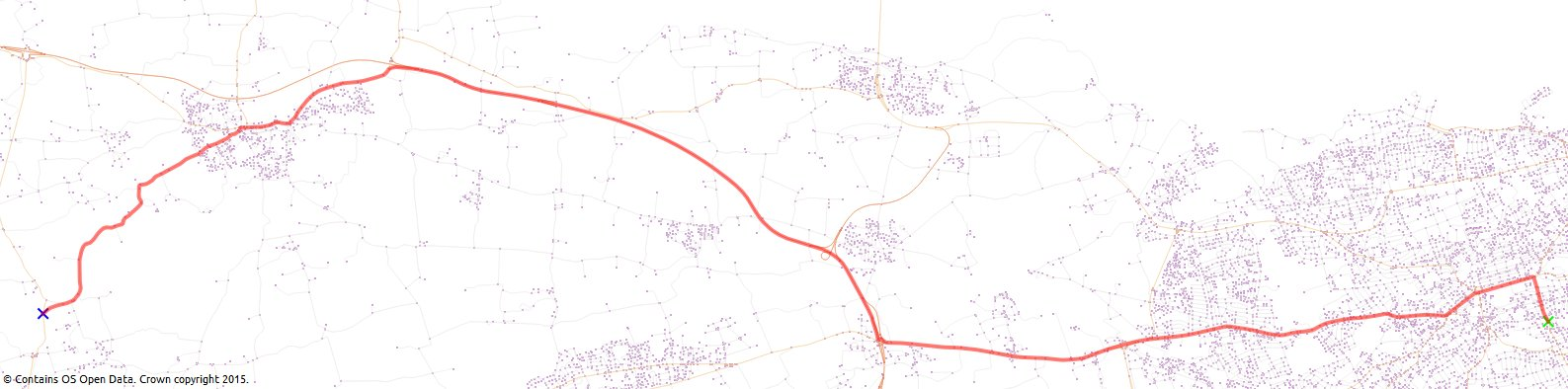Using both QGIS and PgAdmin III to run the queries results in average times of 1:20 per route (I did multiple runs with times between 1:18 and 1:22). There has to be a way to make things faster. If I am only interested in part of the network between my start and end points then can I eliminate the rest of the links from the query? I can, by using a bounding box created using the start and end points.

This is what a standard query looks like using the Djikstra shortest path algorithm:

``````SELECT seq, id1 AS node, id2 AS edge, cost FROM pgr_dijkstra('
SELECT gid AS id,
source::integer,
target::integer,
cost_time::double precision AS cost,
rcost_time::double precision AS reverse_cost
FROM or_network',
100828, 1136587, false, true);
``````

In my network start point 100828 is down in Cornwall and end point 1136587 is near Hull.

Now, we want to add a bounding box to the query to eliminate all the bits of the network we (hopefully) won't need and so make the query faster:

``````SELECT seq, id1 AS node, id2 AS edge, cost FROM pgr_dijkstra('
SELECT gid AS id,
source::integer,
target::integer,
cost_time::double precision AS cost,
rcost_time::double precision AS reverse_cost
FROM or_network
WHERE geometry && ST_Expand(
(SELECT ST_Collect(the_geom) FROM or_network_vertices_pgr WHERE id IN (100828, 1136587)),5000)',
100828, 1136587, false, true);
``````

So what happens here is that the network geometry is filtered using a bounding box created from the start and end points. First, we collect the loose pieces of the network into a single geometry with ST_Collect. Second, we use ST_Expand to return a bounding box expanded in all directions from the single input geometry from ST_Collect. In this example I have expanded the geometry by 5000m (I'm working in British National Grid / EPSG:27700). This parameter can be tweaked because if it is too small your query won't return any results.

Using the bounding box the query result was returned in 27s (still slow) compared to 78s - 86s without it. For points closer to each other and a smaller ST_Expand distance argument the query result was back quicker than you can say "Six sick hicks nick six slick bricks with picks and sticks".

The pgRouting Layer plugin in QGIS uses the stock routing algorithms and could probably be customised to use a bounding box to speed things up.

Using the DB Manager plugin in QGIS allows you to run the routing queries against the database and the load the query results as a new layer. The query above needs a few changes to add in the geometry to display the route on the map. Add the geometry by joining the query result back to the road network:

``````SELECT seq, id1 AS node, id2 AS edge, cost, geometry FROM pgr_dijkstra('
SELECT gid AS id,
source::integer,
target::integer,
cost_time::double precision AS cost,
rcost_time::double precision AS reverse_cost
FROM or_network
WHERE geometry && ST_Expand(
(SELECT ST_Collect(the_geom) FROM or_network_vertices_pgr WHERE id IN (1640725, 1023198)),2000)',
1640725, 1023198, false, true) AS route
JOIN or_network nw
ON route.id2 = nw.gid;
``````

Use the seq column as the unique ID and the geometry column for the geometry.

Bounding box solution above from GIS.SE

#### The Data

Improving the accuracy of the results returned by pgRouting means you need to check the network for sources of potential error. Open Roads has been built so that over and under passes are not noded. This means pgRouting will route correctly over a bridge and not decide to drop onto the road below in an attemp to find the cheapest path. See the image below with the A926 running over the A90 underneath - no nodes.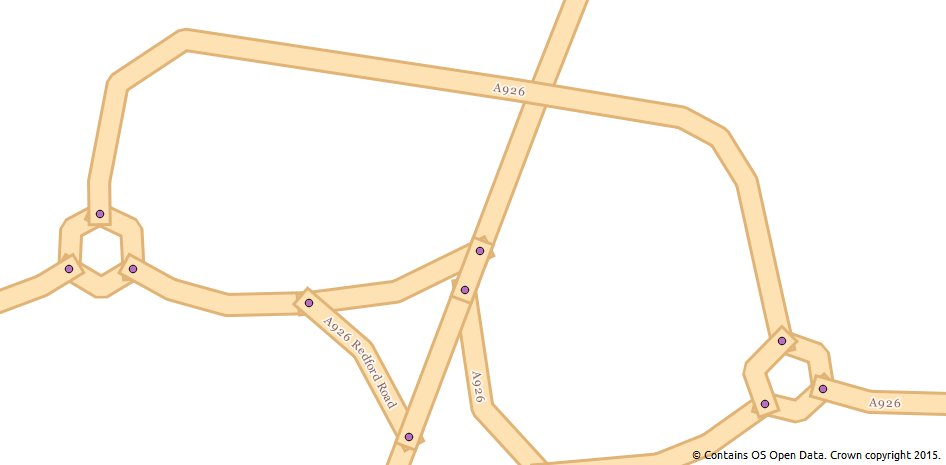#### Sources of error

What does need some working and thinking about is how sections of network that are one-way only are modelled. Open Roads contains a "formofway" attribute and this identifies roundabouts and slip roads. These road links also have a "startnode" and "endnode" attribute which should be able to help define traffic flow direction.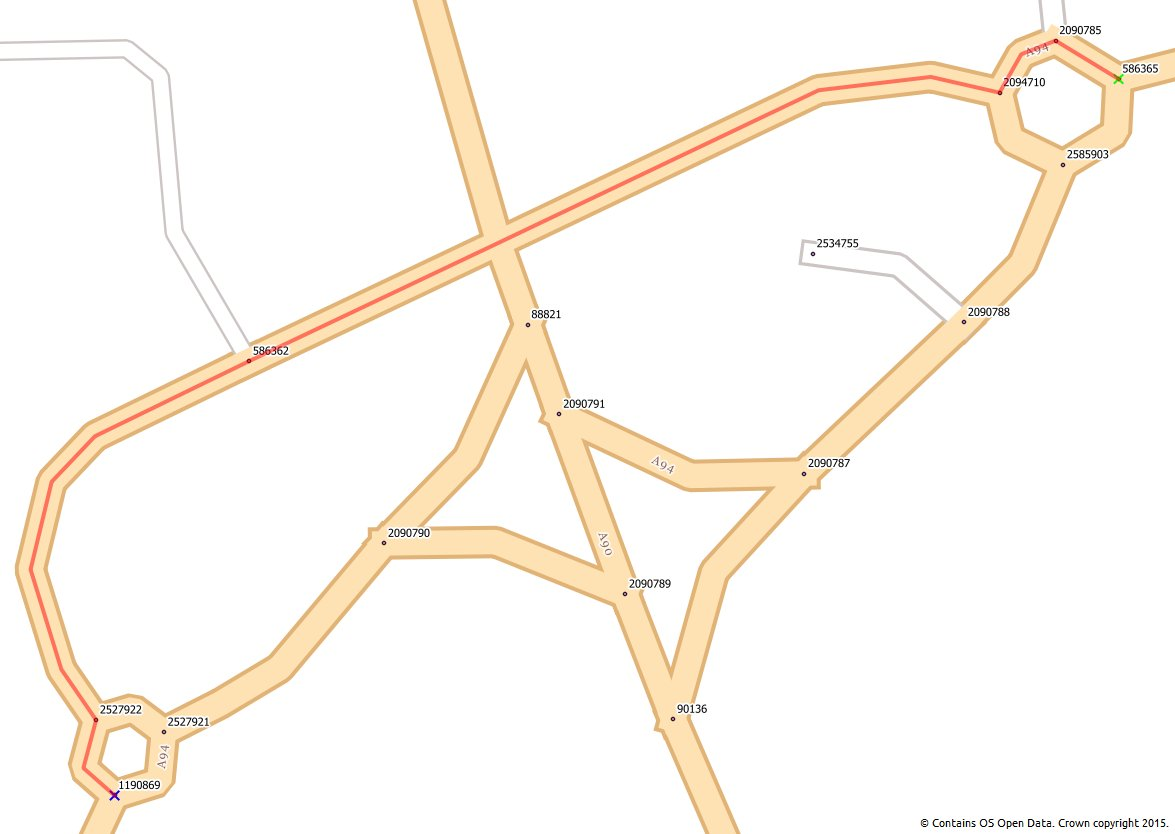Above, this is the correct routing solution using time as the cost.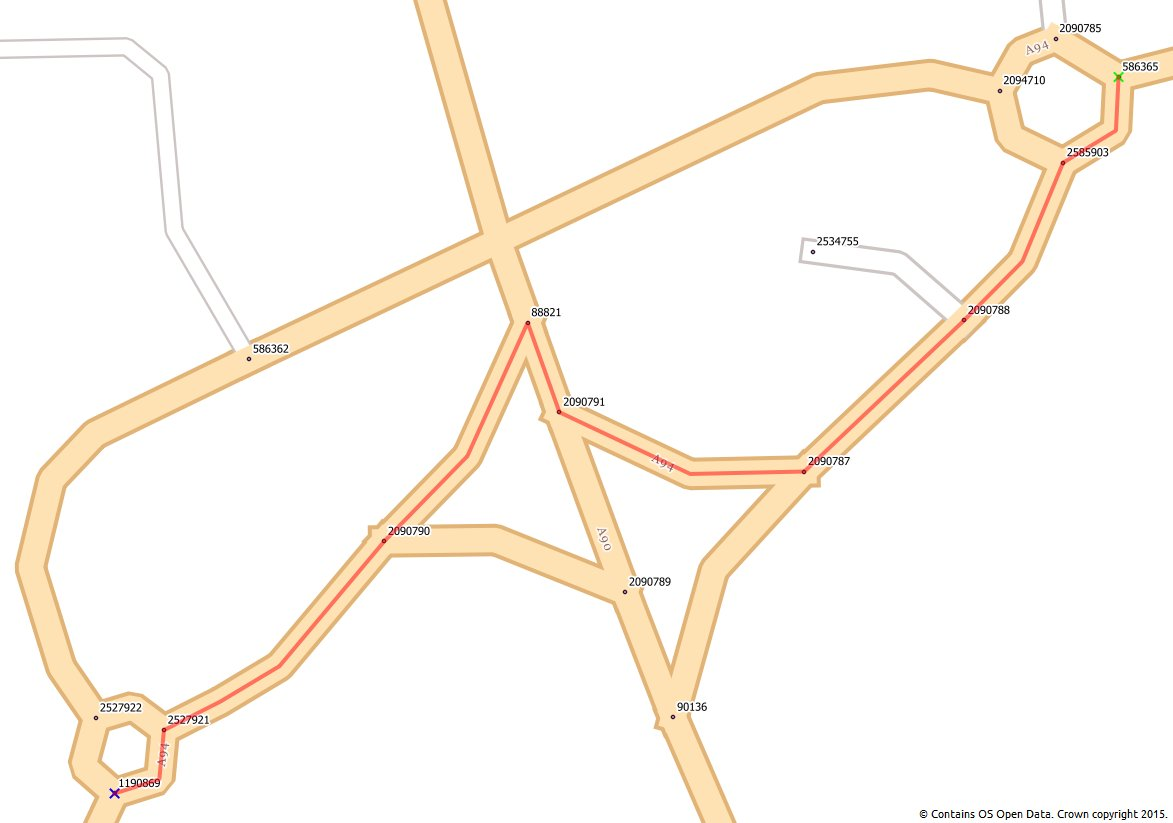Above, this is incorrect using distance as a cost. The route has gone the wrong way around the circle, crossed the collapsed dual carriageway and then around the next circle the wrong way as well. A one-way flag would sort this as links could then be given a high reverse cost value. pgRouting would then route correctly.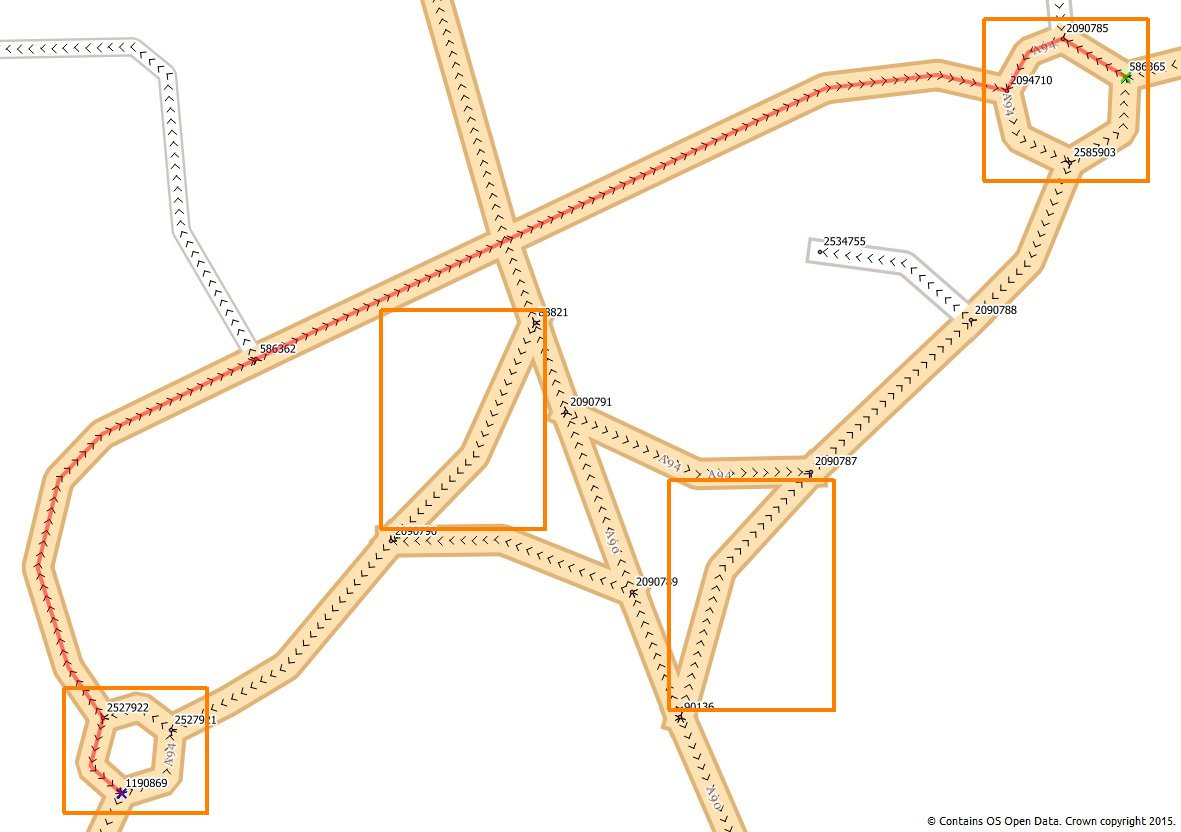Using arrows to show the digitised direction of the lines you can see where circles and slip roads are digitised against the direction of travel. This is not consistent across the dataset and so there is not one rule we can apply to fix the problem. Suggestions welcome!